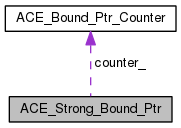ACE  6.0.6
ACE_Strong_Bound_Ptr Class Reference

This class implements support for a reference counted pointer. More...

`#include <Bound_Ptr.h>`

Collaboration diagram for ACE_Strong_Bound_Ptr:[legend]

List of all members.

## Public Member Functions

ACE_Strong_Bound_Ptr (X *p=0)
ACE_Strong_Bound_Ptr (auto_ptr< X > p)
ACE_Strong_Bound_Ptr (const ACE_Strong_Bound_Ptr< X, ACE_LOCK > &r)
Copy constructor binds `this` and r to the same object.
ACE_Strong_Bound_Ptr (const ACE_Weak_Bound_Ptr< X, ACE_LOCK > &r)
Constructor binds `this` and r to the same object.
template<class Y >
ACE_Strong_Bound_Ptr (const ACE_Strong_Bound_Ptr< Y, ACE_LOCK > &r)
~ACE_Strong_Bound_Ptr (void)
Destructor.
void operator= (const ACE_Strong_Bound_Ptr< X, ACE_LOCK > &r)
Assignment operator that binds `this` and r to the same object.
void operator= (const ACE_Weak_Bound_Ptr< X, ACE_LOCK > &r)
Assignment operator that binds `this` and r to the same object.
template<class Y >
ACE_Weak_Bound_Ptr< X, ACE_LOCK > & operator= (const ACE_Strong_Bound_Ptr< Y, ACE_LOCK > &r)
bool operator== (const ACE_Strong_Bound_Ptr< X, ACE_LOCK > &r) const
bool operator== (const ACE_Weak_Bound_Ptr< X, ACE_LOCK > &r) const
bool operator== (X *p) const
bool operator!= (const ACE_Strong_Bound_Ptr< X, ACE_LOCK > &r) const
Inequality operator, which is the opposite of equality.
bool operator!= (const ACE_Weak_Bound_Ptr< X, ACE_LOCK > &r) const
Inequality operator, which is the opposite of equality.
bool operator!= (X *p) const
Inequality operator, which is the opposite of equality.
X * operator-> (void) const
Redirection operator.
X & operator* (void) const
Dereference operator.
X * get (void) const
Get the pointer value.
void reset (X *p=0)
void reset (auto_ptr< X > p)
bool null (void) const

## Public Attributes

ACE_ALLOC_HOOK_DECLARE
Declare the dynamic allocation hooks.

## Private Types

typedef X X_t
typedef ACE_Bound_Ptr_Counter
< ACE_LOCK >
COUNTER
The ACE_Bound_Ptr_Counter type.

## Private Attributes

COUNTERcounter_
The reference counter.
X * ptr_
The underlying object.

## Friends

class ACE_Weak_Bound_Ptr< X, ACE_LOCK >
class ACE_Strong_Bound_Ptr

## Detailed Description

This class implements support for a reference counted pointer.

Assigning or copying instances of an ACE_Strong_Bound_Ptr will automatically increment the reference count of the underlying object. When the last instance of an ACE_Strong_Bound_Ptr that references a particular object is destroyed or overwritten, it will invoke delete on its underlying pointer.

## Member Typedef Documentation

 typedef ACE_Bound_Ptr_Counter ACE_Strong_Bound_Ptr::COUNTER` [private]`
 typedef X ACE_Strong_Bound_Ptr::X_t` [private]`

## Constructor & Destructor Documentation

 ACE_Strong_Bound_Ptr::ACE_Strong_Bound_Ptr ( X * p = `0` ) ` [inline, explicit]`

Constructor that initializes an ACE_Strong_Bound_Ptr to point to the object <p> immediately.

 ACE_Strong_Bound_Ptr::ACE_Strong_Bound_Ptr ( auto_ptr< X > p ) ` [inline, explicit]`

Constructor that initializes an ACE_Strong_Bound_Ptr by stealing ownership of an object from an auto_ptr.

 ACE_Strong_Bound_Ptr::ACE_Strong_Bound_Ptr ( const ACE_Strong_Bound_Ptr< X, ACE_LOCK > & r ) ` [inline]`

Copy constructor binds `this` and r to the same object.

 ACE_Strong_Bound_Ptr::ACE_Strong_Bound_Ptr ( const ACE_Weak_Bound_Ptr< X, ACE_LOCK > & r ) ` [inline]`

Constructor binds `this` and r to the same object.

template<class Y >
 ACE_Strong_Bound_Ptr::ACE_Strong_Bound_Ptr ( const ACE_Strong_Bound_Ptr< Y, ACE_LOCK > & r ) ` [inline]`

Copy constructor binds `this` and r to the same object if Y* can be implicitly converted to X*.

 ACE_Strong_Bound_Ptr::~ACE_Strong_Bound_Ptr ( void ) ` [inline]`

Destructor.

## Member Function Documentation

 X * ACE_Strong_Bound_Ptr::get ( void ) const` [inline]`

Get the pointer value.

 bool ACE_Strong_Bound_Ptr::null ( void ) const` [inline]`

Allows us to check for NULL on all ACE_Strong_Bound_Ptr objects.

 bool ACE_Strong_Bound_Ptr::operator!= ( const ACE_Strong_Bound_Ptr< X, ACE_LOCK > & r ) const` [inline]`

Inequality operator, which is the opposite of equality.

 bool ACE_Strong_Bound_Ptr::operator!= ( const ACE_Weak_Bound_Ptr< X, ACE_LOCK > & r ) const` [inline]`

Inequality operator, which is the opposite of equality.

 bool ACE_Strong_Bound_Ptr::operator!= ( X * p ) const` [inline]`

Inequality operator, which is the opposite of equality.

 X & ACE_Strong_Bound_Ptr::operator* ( void ) const` [inline]`

Dereference operator.

 X * ACE_Strong_Bound_Ptr::operator-> ( void ) const` [inline]`

Redirection operator.

 void ACE_Strong_Bound_Ptr::operator= ( const ACE_Strong_Bound_Ptr< X, ACE_LOCK > & r ) ` [inline]`

Assignment operator that binds `this` and r to the same object.

 void ACE_Strong_Bound_Ptr::operator= ( const ACE_Weak_Bound_Ptr< X, ACE_LOCK > & r ) ` [inline]`

Assignment operator that binds `this` and r to the same object.

template<class Y >
 ACE_Weak_Bound_Ptr& ACE_Strong_Bound_Ptr::operator= ( const ACE_Strong_Bound_Ptr< Y, ACE_LOCK > & r ) ` [inline]`

Assignment operator that binds `this` and r to the same object if Y* can be implicitly converted to X*.

 bool ACE_Strong_Bound_Ptr::operator== ( const ACE_Strong_Bound_Ptr< X, ACE_LOCK > & r ) const` [inline]`

Equality operator that returns `true` if both ACE_Strong_Bound_Ptr instances point to the same underlying object.

Note:
It also returns `true` if both objects have just been instantiated and not used yet.
 bool ACE_Strong_Bound_Ptr::operator== ( const ACE_Weak_Bound_Ptr< X, ACE_LOCK > & r ) const` [inline]`

Equality operator that returns true if the ACE_Strong_Bound_Ptr and ACE_Weak_Bound_Ptr objects point to the same underlying object.

Note:
It also returns `true` if both objects have just been instantiated and not used yet.
 bool ACE_Strong_Bound_Ptr::operator== ( X * p ) const` [inline]`

Equality operator that returns `true` if the ACE_Strong_Bound_Ptr and the raw pointer point to the same underlying object.

 void ACE_Strong_Bound_Ptr::reset ( X * p = `0` ) ` [inline]`

Resets the ACE_Strong_Bound_Ptr to refer to a different underlying object.

 void ACE_Strong_Bound_Ptr::reset ( auto_ptr< X > p ) ` [inline]`

Resets the ACE_Strong_Bound_Ptr to refer to a different underlying object, ownership of which is stolen from the auto_ptr.

## Friends And Related Function Documentation

 friend class ACE_Strong_Bound_Ptr` [friend]`
 friend class ACE_Weak_Bound_Ptr< X, ACE_LOCK >` [friend]`

## Member Data Documentation

Declare the dynamic allocation hooks.

 COUNTER* ACE_Strong_Bound_Ptr::counter_` [private]`

The reference counter.

 X* ACE_Strong_Bound_Ptr::ptr_` [private]`

The underlying object.

The documentation for this class was generated from the following files: